[Introduction]  [Signal arithmetic]  [Signals and noise]   [Smoothing]   [Differentiation]  [Peak Sharpening]  [Harmonic analysis]   [Fourier convolution]  [Fourier deconvolution]  [Fourier filter]  [Wavelets]   [Peak area measurement]  [Linear Least Squares]  [Multicomponent Spectroscopy]  [Iterative Curve Fitting]  [Hyperlinear quantitative absorption spectrophotometry] [Appendix and Case Studies]  [Peak Finding and Measurement]  [iPeak]   [iSignal]  [Peak Fitters]   [iFilter]  [iPower]  [List of downloadable software]  [Interactive tools]

###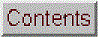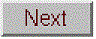Appendix T. Dealing with wide ranging signals: segmented processing functions (added November 2016)

To facilitate the inspection of very large and complex signals, it's useful to be able to "zoom in" to different parts of the x-axis range, which can be done with the interactive Matlab tools iSignal, iPeak, and ipf.m or by the Matlab/Octave function segplot.m. Sometimes an experimental signal will vary so much across its x-axis range that it's impossible to find a single setting for operations like smoothing or peak detection that is optimized for all regions of the signal. It's always possible to break up the signal into pieces and treat each separately, for example using segplot, but in some cases it's easier to use a single segmented application over the entire signal. That's the idea behind the Matlab/Octave functions and the Excel spreadsheet templates in this section.

SegmentedSmooth.m, illustrated on the right, is a segmented variant of fastsmooth.m,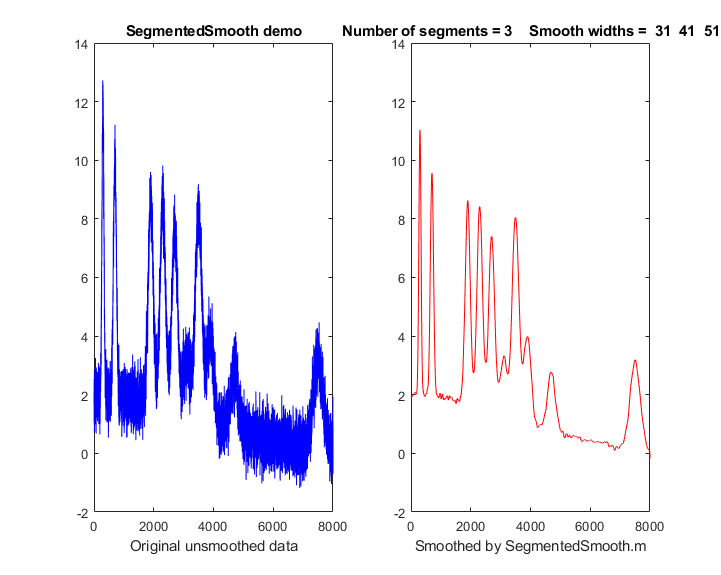which can be useful if the widths of the peaks or the noise level varies substantially across the signal. The syntax is that same as fastsmooth.m, except that the second input argument "smoothwidths" can be a vector: SmoothY = SegmentedSmooth (Y, smoothwidths,type,ends). The function divides Y into a number of equal-length regions defined by the length of the vector 'smoothwidths', then smooths each region with a smooth of type 'type' and width defined by the elements of vector 'smoothwidths'. In the simple example in the figure on the right, smoothwidths=[31 52 91], which divides up the signal into three regions and smooths the first region with smoothwidth 31, the second with 51, and the last with 91. Any number and sequence of smooth widths can be used. If the peak widths increase or decrease regularly across the signal, you can calculate the smoothwidths vector by giving only the number of segments ("NumSegments") , the first value, "startw", and the last value, "endw", like so:

wstep=(endw-startw)/NumSegments;
smoothwidths=startw:wstep:endw;

Type "help SegmentedSmooth" for other examples examples. DemoSegmentedSmooth.m demonstrates the operation with different signals consisting of noisy variable-width peaks that get progressively wider, like the figure on the right. FindpeaksSL.m is the same thing for Lorentzian peaks.

SegmentedSmoothTemplate.xlsx is a segmented multiple-width data smoothing spreadsheet template, functionally similar to SegmentedSmooth.m. In this version there are 20 segments. SegmentedSmoothExample.xlsx is an example with data (graphic). A related spreadsheet GradientSmoothTemplate.xlsx (graphic) performs a linearly increasing (or decreasing) smooth width across the entire signal, given only the start and end values, automatically generating as many segments are are necessary. Of course, as is usual with spreadsheets, you'll have to modify these templates for your particular number of data points, usually by inserting rows somewhere in the middle and then drag-copying down from above the insert, plus you may have to change the x-axis range of the graph. (In contrast, the Matlab/Octave functions do that automatically).

Segmented Fourier filter. SegmentedFouFilter.m is a segmented version of  FouFilter.m, which applies different center frequencies and widths to different segments of the signal. The syntax is the same as FouFilter.m except that the two input arguments 'centerFrequency' and 'filterWidth' must be vectors with the values of centerFrequency of filterWidth for each segment. The signal is divided equally into a number of segments determined by the length of centerFrequency and filterWidth, which must be equal. Type 'help SegmentedFouFilter' for help and examples.

findpeaksSG.m is a variant of the findpeaksG function, with the same syntax, except that the four peak detection parameters can be vectors, dividing up the signal into regions that are optimized for peaks of different widths. Any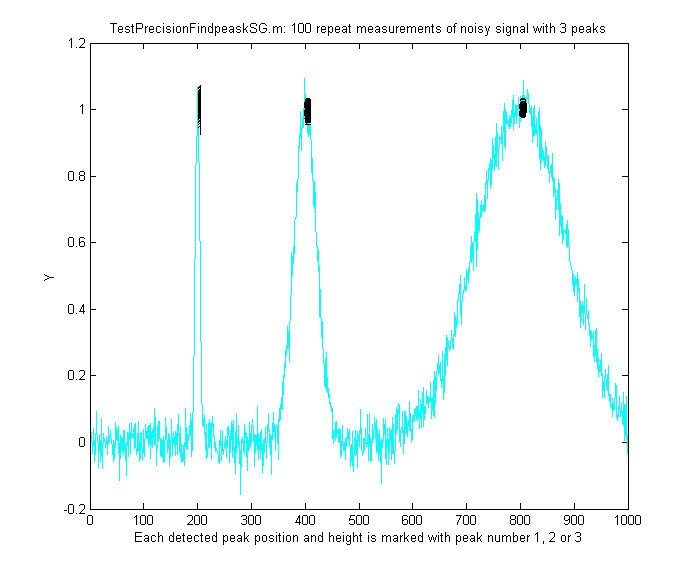number of segments can be declared, based on the lengths of the SlopeThreshold input argument. (Note: you need only enter vectors for those parameters that you want to vary between segments; to allow any of the other peak detection parameters to remain unchanged across all segments, simply enter a single scalar value for that parameter; only the SlopeThreshold must be a vector). In the example shown on the left, the script TestPrecisionFindpeaksSG.m creates a noisy signal with three peaks of widely different widths, measures the peak positions, heights and widths of each peak using findpeaksSG, and prints out the percent relative standard deviations of parameters of the three peaks in 100 measurements with independent random noise. With 3-segment peak detection parameters, findpeaksSG reliably detects and accurately measures all three peaks. In contrast, findpeaksG, tuned to the middle peak (using line 26 instead of line 25), measures the first and last peaks poorly. You can also see that the precision of peak parameter measurements gets progressively better (smaller relative standard deviation) the larger the peak widths, simply because there are more data points in those peaks. (You can change any of the variables in lines 10-18).

findpeaksSb.m is a segmented variant of the findpeaksb.m function.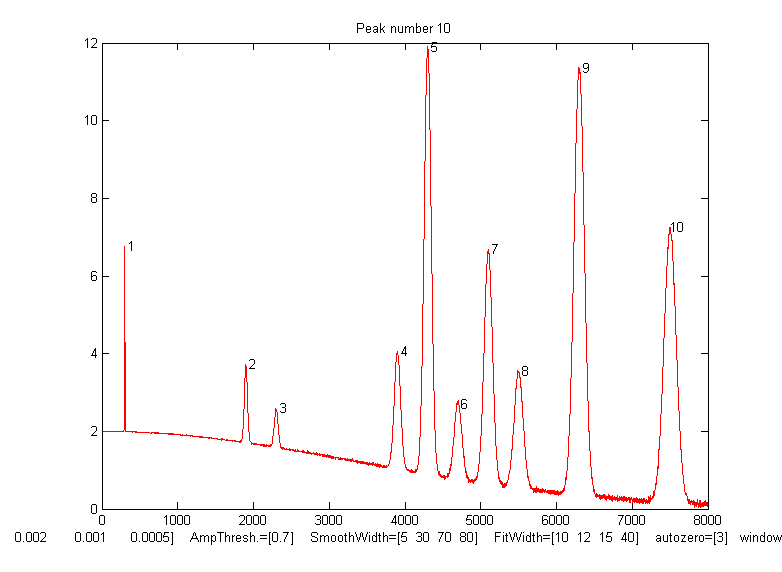It has the same syntax as findpeaksSb, except that the arguments "SlopeThreshold", "AmpThreshold", "smoothwidth", "peakgroup", "window", "PeakShape", "extra", "NumTrials", "autozero", and "fixedparameters" can all be optionally scalars or vectors with one entry for each segment. This allows the function to handle widely varying signals with peaks of very different shapes and widths and backgrounds. In the example on the right, the Matlab/Octave script DemoFindPeaksSb.m creates a series of Gaussian peaks whose widths increase by a factor of 25 and that are superimposed in a curved baseline with random white noise that increases gradually across the signal. In this example, four segments are used, changing the peak detection and curve fitting values so that all the peaks are measured accurately.

SlopeThreshold = [.01 .005 .002 .001];
AmpThreshold = 0.7;
SmoothWidth = [5 15 30 35];
FitWidth = [10 12 15 20];
windowspan = [100 125 150 200];
peakshape = 1;
autozero = 3;

The script also computes the relative percent error of the measurement of peak position, height, width, and area for each peak.measurepeaks.m is an automatic peak detector peaks of arbitrary shape. It is based on findpeaksSG, with which it shares the first 6 input arguments; the four peak detection arguments can be vectors to accommodate signals with peaks of widely varying widths. It returns a table containing the peak number, peak position, absolute peak height, peak-valley difference, perpendicular drop area, and tangent skim area of each peak it detects. If the last input argument ('plots') is set to 1, it will plot the entire signal with numbered peaks and will also plot the individual peaks with the peak maximum (red), valley points (magenta), and tangent lines (cyan), as shown on the right. The peak height and x-position is indicated by the red circle, the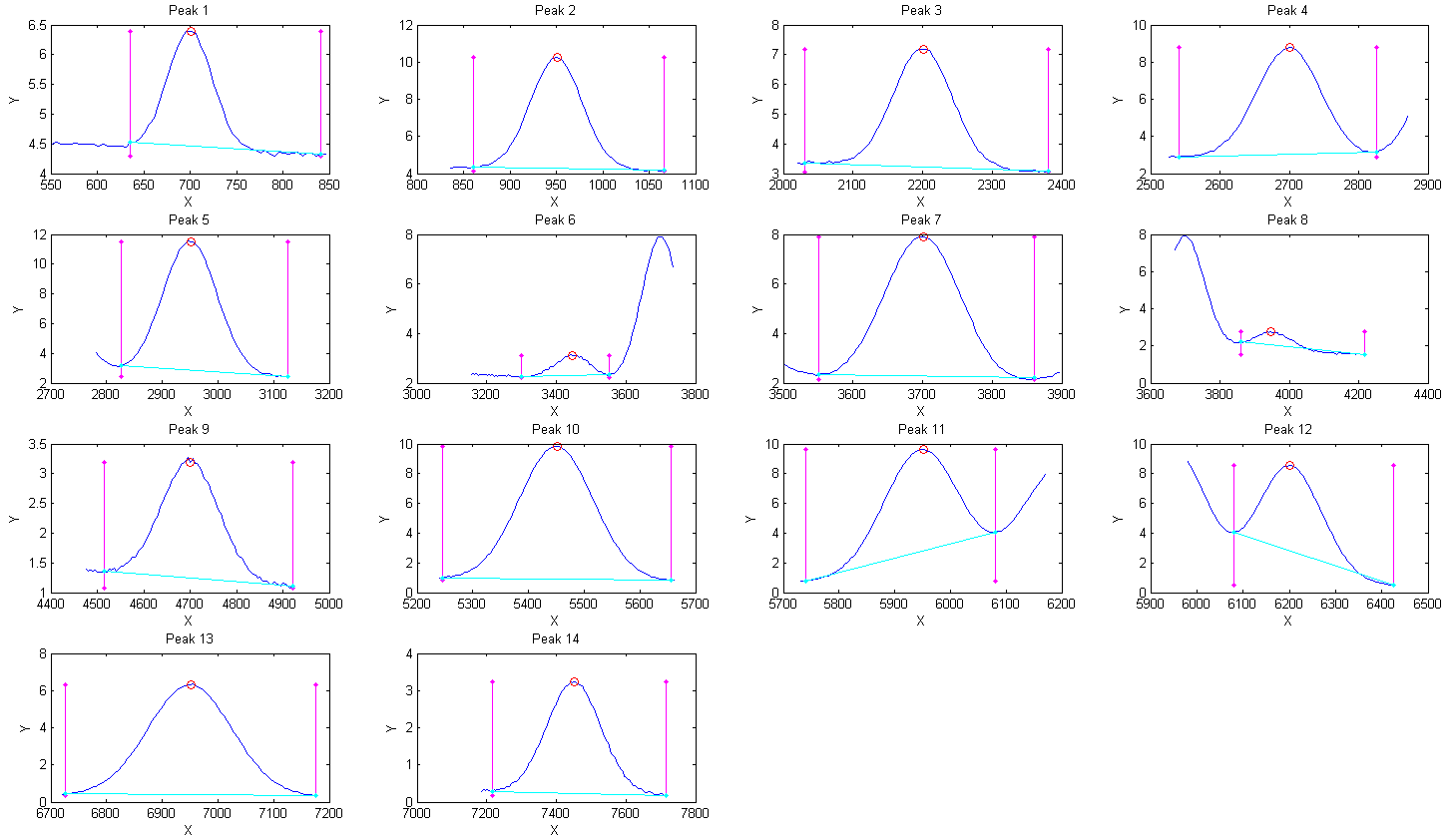perpendicular drop area is the total area measured between the two magenta vertical lines down to zero, and the tangent skim area is the area between the cyan baseline and the blue peak (which compensates for a flat baseline). Type "help measurepeaks" and try the examples there or run testmeasurepeaks.m (graphic animation). The related functions autopeaks.m and autopeaksplot.m are similar, except that the four peak detection parameters can be omitted and the function will calculate estimated initial values.

The script HeightAndArea.m tests the accuracy of peak height and area measurement with signals that have multiple peaks with variable width, noise, background, and peak overlap. Generally, the values for absolute peak height and perpendicular drop area are best for peaks that have no background, even if they are slightly overlapped, whereas the values for peak-valley difference and for tangential skim area are better for isolated peaks on a straight or slightly curved background. Note: this function uses smoothing (specified by the SmoothWidth input argument) only for peak detection; it performs its measurements on the raw unsmoothed y data. If the raw data are noisy, it may be best to smooth the y data yourself before calling measurepeaks.m, using any smooth function of your choice.

Other segmented functions. The same segmentation code used in SegmentedSmooth.m (lines 53-65) can be applied to other functions simply by editing the first line in the first for/end loop (line 59) to refer to the function that you want to apply in a segmented fashion. For example, segmented peak sharpening can be useful when a signal has multiple peaks that vary in width, and segmented deconvolution can be useful when a signal has multiple peaks that vary in width or tailing vary substantially across the signal: SegExpDeconv(x,y,tc) deconvolutes y with a vector of exponential functions whose time constants are specified by the vector tc. SegExpDeconvPlot.m is the same except that it plots the original and deconvoluted signals and shows the divisions between the segments by vertical magenta lines.

This page is part of "A Pragmatic Introduction to Signal Processing", created and maintained by Prof. Tom O'Haver , Department of Chemistry and Biochemistry, The University of Maryland at College Park. Comments, suggestions and questions should be directed to Prof. O'Haver at toh@umd.edu. Updated July, 2022.